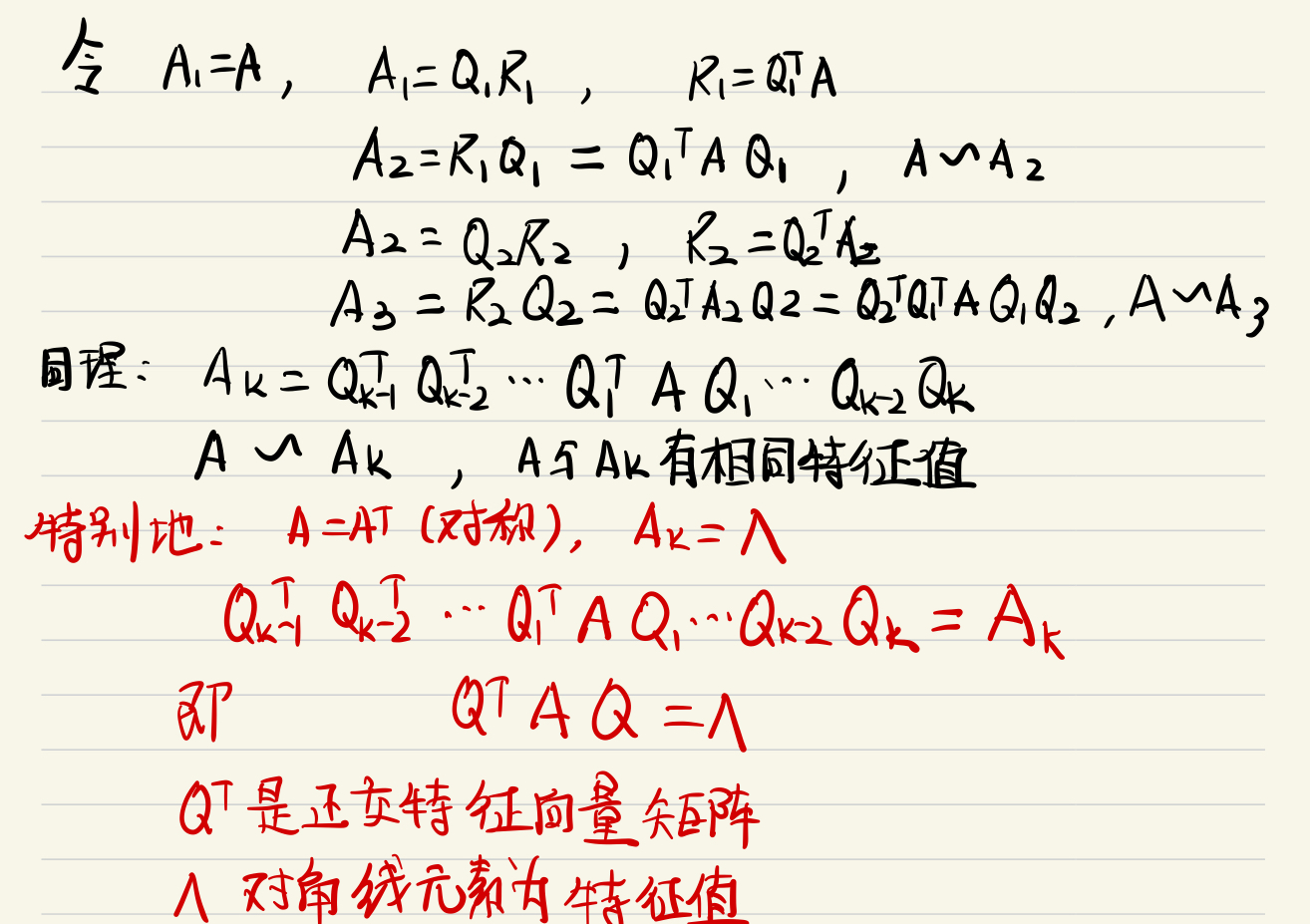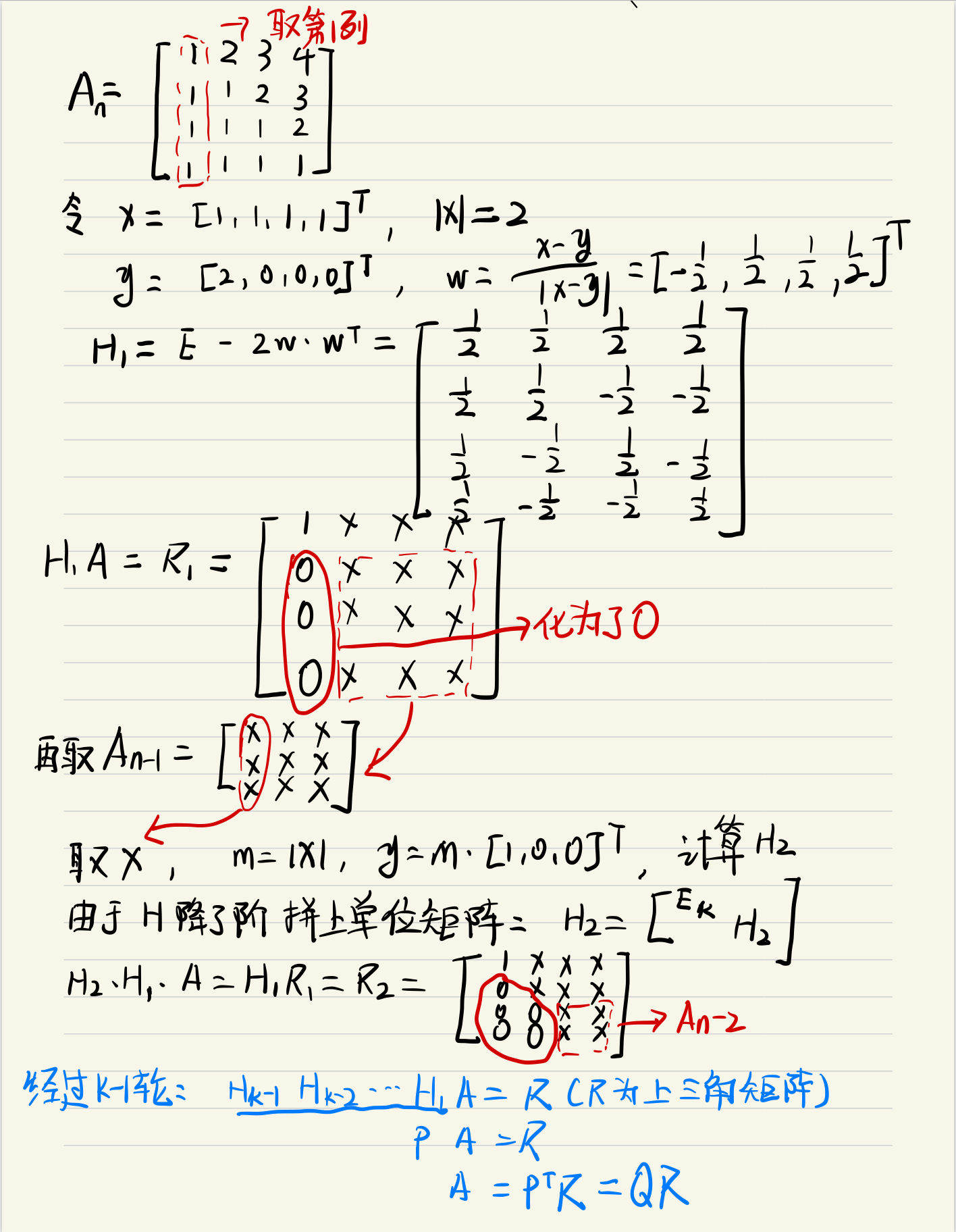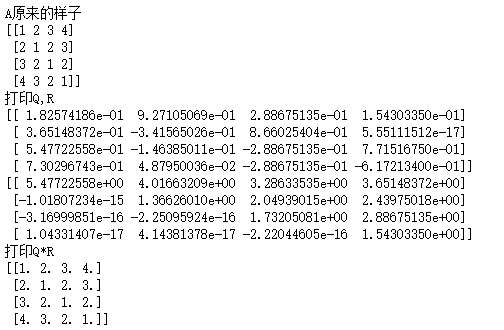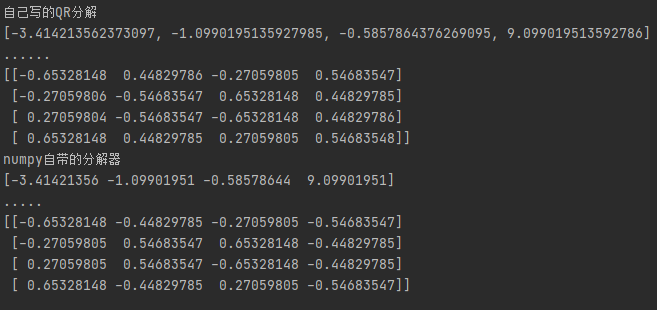• 特征值 特征向量 广义特征值 matlab
千次阅读
2021-04-20 02:52:56

d=eig(A) www.iLoveMatlab.cn

返回矩阵A特征值的一个向量d。

d=eig(A,B)

www.iLoveMatlab.cn

如果A和B是方阵的，返回包含广义特征的向量。

注意：如果S是稀疏对称的，用d = eig(S) 可以返回S的特征值。如果S是稀疏但不对称，若要想得到S的特征向量，用函数eigs代替eig。

[V,D]=eig(A) book.iLoveMatlab.cn

计算矩阵A的特征值D和特征矩阵V，满足A*V = V*D。矩阵D是矩阵A的规范形式：主对角线上的元素是矩阵A的特征值的对角矩阵。

[V,D] = eig(A,'nobalance')

当矩阵A中有与截断误差数量级相差不远的值时，改命令可能更加精确，'nobalance'起误差调节作用。

[V,D] = eig(A,B)

计算广义特征向量矩阵V和广义特征值矩阵D，满足A*V = B*V*D 。

[V,D] = eig(A,B,flag)

由flag指定算法来计算特征值D和特征向量V，flag的可能值为：

'chol'：表示对B进行cholesky分解算法，这里A是对称的Hermitian矩阵，B为正定矩阵。 Matlab中文论坛

'qz'：表示使用qz分解算法，这里A、B是非对称或非Hermitian矩阵。

广义特征值是求解Ax=λBx的非0解,满足的λ值。当A,B是方阵就可以用eig(A,B)求解

特征值是求解Ax=λx的非0解,满足的λ值。当A是方阵 则可用eig(A)求解

1. eig(pinv(A)*B) 是求解 pinv(A)*Bx=λx 就是 A-1Bx=λx

2. eig(A,B)  是求解 Ax=λBx  就是 B-1Ax=λx

在MATLAB中，计算矩阵A的特征值和特征向量的函数是eig(A)，常用的调用格式有

5种：

(1) E=eig(A)：求矩阵A的全部特征值，构成向量E。

(2) [V,D]=eig(A)：求矩阵A的全部特征值，构成对角阵D，并求A的特征向量构成

V的列向量。

(3) [V,D]=eig(A,'nobalance')：与第2种格式类似，但第2种格式中先对A作相似

变换后求矩阵A的特征值和特征向量，而格式3直接求矩阵A的特征值和特征向量。

(4) E=eig(A,B)：由eig(A,B)返回N×N阶方阵A和B的N个广义特征值，构成向量E

(5) [V,D]=eig(A,B)：由eig(A,B)返回方阵A和B的N个广义特征值，构成N×N阶对

角阵D，其对角线上的N个元素即为相应的广义特征值，同时将返回相应的特征向

量构成N×N阶满秩矩阵，且满足AV=BVD。

更多相关内容
• 使用c语言求矩阵的特征值和特征向量。使用数值积分方法，纯c语言编写，主要是用的QR分解，vs工程文件。
• 主要为大家详细介绍了C++ Eigen库计算矩阵特征值及特征向量，具有一定的参考价值，感兴趣的小伙伴们可以参考一下
• 特征值与特征向量定义 Ax =λx,则λ是A的一个特征值,x为A对应于特征值λ的特征向量 特征值为零，矩阵不可逆 求A的特征值:解特征多项式det(A-λI) eigen求矩阵的特征值与特征向量,但有限制，必须是对称矩阵 #include ...
• 在使用AHP(层次分析法)时，需要计算特征值，使用pyhon读取Excel数据处理 此程序使用python读excel数据计算特征值和特征向量； 使用了numpy、xlrd、xlwt库；
• 纯C语言实现eig算法，通过C语言求解矩阵的特征值与特征向量，主要用到QR分解。结果与matlab结果对比相同
• 利用第三方矩阵计算工具包JAMA，求特征值、特征向量 API： http://math.nist.gov/javanumerics/jama/doc/Jama/Matrix.html 应用： 1.JAMA包的导入 1）https://mvnrepository.com/ 2）搜索JAMA，选第一个：gov....
• 深入整理了雅克比方法求解特征值和特征向量，包括公式推导，最后介绍了opencv中的算法流程和实现。
• 第二十四篇 特征值问题编程基础：特征值特征向量的求解和性质 特征值方程 在分析结构稳定性或振动系统的固有频率时，经常会出现这种情况。我们必须找到一个向量{x}，当它与[a]相乘时，得到它自身的标量倍。这个λ...

# 第二十四篇 特征值问题编程基础：特征值特征向量的求解和性质

## 特征值方程

在分析结构稳定性或振动系统的固有频率时，经常会出现这种情况。我们必须找到一个向量{x}，当它与[a]相乘时，得到它自身的标量倍。这个λ倍数称为[A]的“特征值”，我们将看到n阶矩阵有n个这样的λ。从物理上讲，它们可能代表振荡的频率。还有与每个特征值λ相关的n个向量{x}。这些被称为“特征向量”。从物理上讲，它们可能代表振荡的模态振型。下面是一个具体的例子：重新排列后可以写成下面的形式这组线性联立方程只有在系数的行列式为零时才可能有非0解行列式展开得这叫做“特征多项式”。显然，解特征值方程的一种方法是将其简化为一个n次特征多项式，并使用前一章的方法来求其根。有时这样做，也许作为整个解过程的一部分，但它本身通常不是求解特征值方程的最佳方法。
上面得特征多项式有一个简单得因式分解所以特征值解为4，1，-8
对于任意矩阵[A]，特征多项式都可能产生虚根和实根。所以要把问题限制在具有实数特征值的矩阵上，物理约束通常意味着矩阵是对称的和“正定的”，在这种情况下，所有的特征值都是实数和正数。找到一个特征值后，其相关的特征向量可以通过求解一组线性联立方程来找到。例如，将λ = 1代入方程得到经过高斯消元得第一个阶段由上面可以看出，行列式得值为0，方程数比未知数数量少，可见方程组是线性相关的。然而，我们可以从上面的方程第二行和第三行看出，x2与x3的比值是2:1，通过代换方程的第一行，x1与x2的比值是1:1。所以当特征值λ = 1时，任意一个特征向量的比值x1: x2: x3 = 2:2:1。类似的操作也可以用来求两个特征值对应的特征向量。

## 特征向量的正交化和规范化

特征向量有一个独特的方向，但它们的大小是任意的。两边同时乘以一个任意常数α，得到。在该修正方程中，α{x}和{x}是数组[A]中对应特征值λ的等效特征向量。两种常用的特征向量正交化方法。对于下面的对称矩阵具有特征值对应的特征向量特征向量正交化的一种简便形式包括缩放长度，或者称为“L2范数”或“欧几里德范数”。因此对于一个特征向量的n个分量，我们像下面这个缩放这个向量它是累加全部分量的平方和再求跟得到的
因此，对于第一个特征向量，将除以下面这个长度值对于其他两个特征向量，得到正交特征向量为对称矩阵的特征向量互相具有“正交性”。也就是说，来自同一个矩阵的任意两个不同特征向量的点积等于零，而特征向量与自身的点积为其长度的平方。因此，将欧几里德范数设为单位1的一个便利的特点是是特征向量点积要么等于零，要么等于单位1。特征向量正交化的一种简单的替代形式是除以具有最大(绝对)幅度的分量。得到了一个最大分量等于单位1的正交化特征向量。对于之前给出的特征向量，第一个特征向量除以√2，第二个特征向量不变，因第三个特征向量将被-√2整除得到正交化向量。## 特征值和特征向量的性质

本部分所描述的数值方法利用了很多矩阵变换对特征值和特征向量影响的定理。下面总结了这些关系中使用较多的一些。

### 如果一个n × n矩阵[A]具有实数特征值λ1， λ2，···，λn， [I]为单位矩阵，p为标量位移，则### n × n矩阵对角线的和称为“迹”，也等于特征值的和，因此### 一个n×n矩阵的特征值的乘积等于这个矩阵的行列式，因此### 给定两个方阵[A]和[B]。

[A][B]和[B][A]将有相同的特征值。
如果{x}是[A][B]的特征向量，则[B]{x}是[B][A]的特征向量。
解释：然后两边都乘以B得到### 给定两个方阵[A]和[P]，其中[P]是非0的。

[A]和[P]−1[A][P]具有相同的特征值。
如果{x}是[A]的特征向量，那么[P]−1{x}是[P]−1[A][P]的特征向量。
解释：通过两边都乘以[P]−1，得到嵌入矩阵形式[P][P]-1=[I]，得到### 如果[A]是一个n×n矩阵，其特征值和特征向量为λ1{x1}， λ2{x2}，···，λn{xn}。[P]是一个矩阵，其列是[a]的使其欧几里德范数等于单位的特征向量缩放，那么展开全文• 经典jacobi法求解特征值特征向量，MATLAB代码实现。。MATLAB
• 当Ak基本收敛到为上三角矩阵时，迭代完成，此时主对角元素就是特征值。 特别地：当A是对称阵的时候，Ak是对角阵Λ，Q=Qk-1Qk-2…Q1就是其正交特征向量矩,有QTAQ=Ak=Λ，即A正交对角化与Ak。 如何理解？我们看下图...

# 一 QR原理

理论依据：任意一个非奇异矩阵（满秩的方阵）A都可以分解为一个正交矩阵Q和一个上三角矩阵R的乘积，且当R对角元符号确定时，分解是唯一的。QR分解是一种迭代方法，迭代格式如下：当Ak基本收敛到为上三角矩阵时，迭代完成，此时主对角元素就是特征值。

特别地：当A是对称阵的时候，Ak是对角阵Λ，Q=Qk-1Qk-2…Q1就是其正交特征向量矩,有QTAQ=Ak=Λ，即A正交对角化与Ak。

如何理解？我们看下图公式:所以，QR迭代过程从数学的角度来想其实就是不断正交化的过程。

# 二 QR算法步骤

## 1.Householder变换进行QR分解

反射矩阵：任取单位向量w,反射矩阵H=E-2WWT ,显然HHT =E，H是正交阵

定理：任取两个模长相等的的向量x,y，一定存在一个反射矩阵H，使得Hx=y，
此时w=(x-y)/(|x-y|)（向量的差除以向量差的模）

应用：现在我们取矩阵的一列为x,m=|x|,y=m*[1,0,0,…0]T 根据上面的定理求出H，使得Hx=y,是不是通过正交变化就把那一列化成了[m,0,0,0]T ,这样就达到了将下三角元素全化为0的效果。看下图，举个例子来说明QR分解过程：看懂上述过程就知道，Householder变换是利用了反射定理，经过n-1轮正交变换，将下三角元素全部化为0，从而得到上三角矩阵R，将所有H矩阵左乘运算再转置得到正交矩阵Q，即A=QR

我们看看QR分解的代码：

#QR分解
def qrSplit(A):
n=A.shape#A的维度
Q=[[]]
R=A
for i in range(0,n-1):
B=R
if i!=0:
#删除一行一列,得n-1阶子阵
B=B[i:,i:]
#取第一列向量
x=B[:,0]
#向量摸长
m=np.linalg.norm(x)
#生成一个模长为m，其余项为0的向量y
y=[0 for j in range(0,n-i)]
y=m
#计算householder反射矩阵
#w = (x-y)/||x-y||
w=x-y
w=w/np.linalg.norm(w)
#H=E-2*WT*W
H=np.eye(n-i)-2*np.dot(w.reshape(n-i,1),w.reshape(1,n-i))#H是个正交矩阵
#第一次计算不需对正交正H升维
if i==0:
#第一次迭代
Q=H
R=np.dot(H,R)
else:
#因为降了维度，所以要拼接单位矩阵
D=np.c_[np.eye(i),np.zeros((i,n-i))]
H=np.c_[np.zeros((n-i,i)),H]
H=np.r_[D,H]
#迭代计算正交矩阵Q和上三角R
Q=np.dot(H,Q)
R=np.dot(H,R)
Q=Q.T
return [Q,R]


我们测试一下QR分解后是否能还原：

A=np.array([1,2,3,4,2,1,2,3,3,2,1,2,4,3,2,1])
A=A.reshape(4,4)
print('A原来的样子')
print(A)
qr = qrSplit(A)
print('打印Q,R')
print(qr)
print(qr)
print('打印Q*R')
print(np.dot(qr,qr))很明显，R下三角元素都非常小，可以认为是0.

# 2.QR迭代

上一步完成了QR分解，QR迭代就非常简单了，看代码：


#QR迭代求特征值特征向量
def  qrEgis(A):
# QR迭代(尽量让它多迭代几次，以至于AK收敛为上三角)
qr = []
n = A.shape  # A的维度
Q = np.eye(n)
for i in range(0, 100):
# A=QR
qr = qrSplit(A)
# 将Q右边边累成
Q = np.dot(Q,qr)
# A1=RQ
A = np.dot(qr, qr)

AK = np.dot(qr, qr)
#把e取出来
e=[]
for i in range(0,n):
e.append(AK[i][i])
#对特征值按升序排序，特征向量与其对应
for i in range(0,n-1):
min=e[i]
for j in range(i+1,n):
if e[j]<min:
min=e[j]
#交换特征值
tmp=e[i]
e[i]=e[j]
e[j]=tmp
#交换特征向量
r=np.copy(Q[:,i])
Q[:,i]=Q[:,j]
Q[:,j]=r;
return [e,Q]


我们输入一个对称阵，同时去求出它的特征值与特征向量，测试一下。
并且与numpy自带的求解特征值、特征向量的做个对比，看代码：

A=np.array([1,2,3,4,2,1,2,3,3,2,1,2,4,3,2,1])
A=A.reshape(4,4)
egis =qrEgis(A)
print('自己写的QR分解')
print(egis);
print('......')
print(egis);
print('numpy自带的分解器')
e,u=np.linalg.eigh(A);
print(e)
print('.....')
print(u)可以看出自己写的QR分解法和numpy自带的分解器求出的特征值是一样的。
特征向量在数值上一样，符号不一样并没有关系，因为X和-X都是A的特征向量。

注意：如果A不是对称阵，QR法只能求出全部特征值，不能同时求出特征向量。
但是，已知特征值求特征向量可以采用反幂法。
关于反幂法，学过数值分析的知道，其实也是一个迭代过程。

展开全文机器学习 python
• AHP层次分析法计算权重、特征值、特征向量，第一作者刘兴，其他地方下载需要47个币 【 尚有疑问，欢迎沟通！！ 1.CSDN上有人说可用； 2.亲自试验，代码流畅，但个人感觉特征值、特征向量存疑； 3.用java求出的特征...
• Jacobi法求特征值特征向量引言Jacobi法求特征值特征向量 引言 参考链接1 参考链接2 参考链接3 参考链接4 Jacobi法求特征值特征向量 求解使用对象：实对称矩阵RRR,性质有RR−1=IRR^{-1}=IRR−1=I 即是 RT=R−1R^T=R...

## Jacobi法求特征值特征向量

求解使用对象：实对称矩阵 R R ,性质有 R R − 1 = I RR^{-1}=I 即是 R T = R − 1 R^T=R^{-1}
求解思路：实对称矩阵性质与旋转矩阵 R R 性质相同，故看做是将旋转矩阵转化为三个自由度。变换过程也是利用旋转矩阵相乘来达到。化为对角线性的过程就是旋转的过程，对角线上的元素就是特征值，用来变换的旋转矩阵的列向量就是特征向量。
用处蛮多的：对于协方差矩阵、海塞矩阵的近似 J T J J^TJ 、旋转矩阵的分解均可以使用此方法，精度高，速度快，过程不涉及求逆。分解后的形式： A = V Λ V T A = V \Lambda V^T ，没有求逆，在后续计算也比较方便。
名字为什么叫 J a c o b i Jacobi 法没有深入。

展开全文• 幂法求矩阵特征值特征向量matlab程序，但是区别于matlab自带方法。
• Jacobi方法求解特征值特征向量，Jacobi方法求解特征值特征向量MATLAB
• 特征值特征向量到方向分析（标准差椭圆） 一． 特征值与特征向量的意义                      Ax=λx    几何直观解释为x向量在矩阵A作用下使得x向量方向不变，且拉伸了λ倍。那x向量和λ的具体...
• QR法求解特征值特征向量(MATLAB)课程设计,已经调试成功，里面有4份不同的课设MATLAB
•文档
• QR法求解特征值特征向量(MATLAB)课设,已经调试成功MATLAB
•矩阵 矩阵对角化
• 通过用vba求矩阵的特征值与特征向量。可用于大多数
• 颜庆津版数值分析编程作业，C语言(少量C++语法)实现矩阵的QR分解法迭代求矩阵的全部复数格式特征值，先对矩阵进行拟上三角化再迭代，迭代求出全部特征值后使用列主元素高斯消元法求出所有实特征值对应的特征向量
• 本代码用C语言进行编程，可以计算矩阵的特征值和相应的特征向量
• 雅克比法求取矩阵特征值和特征向量 考虑线性方程组Ax = b时，一般当A为低阶稠密矩阵时，用主元消去法解此方程组是有效方法。但是，对于由工程技术中产生的大型稀疏矩阵方程组，利用迭代法求解此方程组就是合适的，在...
• 已知随机变量X的协方差矩阵求去X的特征值 特征向量 PCA投影矩阵 相关的知识都忘记了，去查的时候没有耐心看别人长篇大论讲解，就只简单记录了一下如果从协方差矩阵来计算特征值和特征向量。 定义：1.特征值...PCA
• 《矩阵特征值与特征向量的定义与性质》教学设计.pdf
• 这几天做一个项目，需要用到 求矩阵的特征值特征向量。我c++学的不好，所以就去网站找了很多java的源代码，来实现这个功能。很多都不完善，甚至是不准确。所以自己参考写了一个。这个用于我一个朋友的毕业设计。结果...
• 雅可比法用于对称矩阵，QR用于非对称...QR分解法求特征向量及其特征值c++程序源于feitian_feiwu的资源，本资源增加了复特征值对应的特征向量计算。 vs2013下调试通过，复特征向量不唯一，程序里提供了在线验证网址，
• ## 特征值与特征向量

万次阅读 2019-08-23 16:58:11
本文参考《Linear Algebra and Its Applications》——David C.Lay, Steven R....A为n*n的矩阵，x为非零向量，若存在数λ使Ax=λx有非平凡解x，则称λ为A的特征值，x称为对应于λ的特征向量。 例：设，......